Next: Signature-splitting Up: Superdeformed bands in S Previous: Energies

Using the same symbols as those introduced in Fig. 6, in Fig. 8 are plotted the proton quadrupole moments, in the form of trajectories of points on the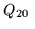-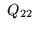plane, corresponding to consecutive values of the rotational frequency. In order to visualize the fact that values of Q22 are always much smaller than those of Q20(small non-axiality), the lines corresponding to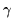=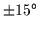and=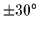, where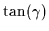=Q22/Q20, are also shown in the figure.
From Fig. 8 it is clearly seen that bands calculated in the present study represent fairly distinct regions of deformation. In order to better visualize the magnitude of the deformation, one can use the simplest first-order formula ,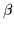=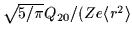), relating the proton axial quadrupole moment with the standard deformation parameter. For the 3232configuration this gives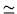Q20/(2.53eb)0.7. Since at the same time the axial hexadecapole moment is fairly small, Q400.06eb2, the first-order formula should be a good estimate of the exact result, corresponding to the deformations of an equivalent sharp-edge uniform charge distribution that has all multipole moments equal to the ones calculated microscopically.
It follows that the ground-state band 3030reaches quadrupole deformationsof the order of 0.16, the intermediate-deformation configurations 3131 correspond to0.45, while the strongest deformed band 3434 has0.8. (The latter band carries, however, Q400.54eb2, and thus the simple one-parameter formula forcan be less precise here.) Results presented in Fig. 8 show a clear correlation of quadrupole moments and numbers of intruder states occupied in a given configuration; we discuss this question in more detail in Sec. 5.